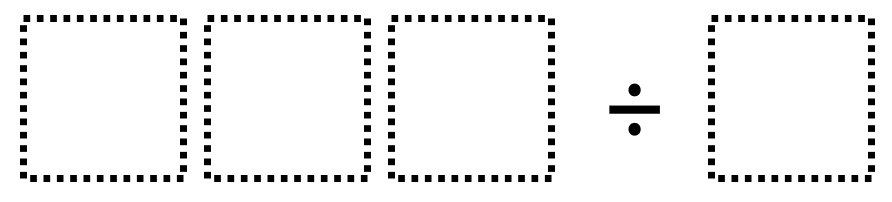# Multi-Digit Division 1

Directions: Using the digits 1 through 9 at most one time each, place a digit in each box to create two different whole number quotients: one that is greater than 300 and one that is less than 300. You may reuse all the digits for each quotient.### Hint

How can we tell if a quotient will have a whole number quotient without dividing? How can we predict whether the quotient will be greater than 300 or less than 300 without actually dividing?

There are many possible answers including 986 ÷ 2 which has a quotient of 493, and 486 ÷ 2, which has a quotient of 243.

Source: Robert Kaplinsky

## Product Close to 1,000

Directions: Using the digits 1 to 9 at most one time each, place a digit …

### One comment

1.One student found what might be the largest quotient 987/1=987.

Another student found what might be the smallest quotient 126/9=14